Mathematics

# Cubic Function

162 views

 1 Introduction 2 Cubic Function 3 Cubic function Domain and Range 4 Inverse of Cubic Function 5 Cubic Function graph 6 How to factor a cubic Function 7 Properties of The Cubic Function 8 Summary 9 FAQs

15 October 2020

## Introduction

Hello Everyone, My Name is Neha Tyagi. Once again I am here with a new topic which is Cubic Function.

What is the meaning of a cubic equation and how such an equation can be solved? The policy for solving a cubic equation is to reduce it to a quadratic equation, and then solve the quadratic by the usual means, either by factoring or using the formula.

## Cubic Function

In mathematics, a cubic function is a function of the form below mentioned.

$f{x}=ax^3+bx^2+cx+d$

Where a ≠ 0

And the coefficients a, b, c, and d are real numbers, and the variable x takes real values. or we can say that it is both a polynomial function of degree three and a real function.

Set $$f (x) = 0,$$ generate a cubic polynomial of the form

$ax^3+bx^2+cx+d$

whose solutions are called roots of the cubic function.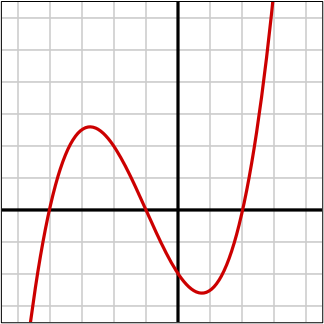All cubic polynomials have one real root, or they have three real roots and all odd-degree cubic polynomials have at least one real root.

All roots of the cubic polynomial can be found by the following means:

• It may be brief by a cubic formula involving the four coefficients and the four basic arithmetic operations and the nth roots.
• Trigonometrically
• Numerical approximation of the roots can be found using root-finding algorithms such as Newton’s Method.

### Cubic Polynomial

A cubic polynomial is also known as a polynomial of degree 3. A univariate cubic polynomial has the form $$f(x)=a_3x^3+a_2x^2+a_1x+a_0$$. A function involving a cubic polynomial is called a cubic function. A closed equation solution is also called the cubic formula exists for the solutions of an arbitrary cubic equation.

## Cubic Function Domain and Range

We have one way to find out the domain and range of cubic functions that is by using graphs. Because the domain is the combination of available input values, the domain of a cubic function graph consists of all the input values shown on the x-axis. And the range of the set of possible output values, which are shown on the y-axis.

When the cubic function graph will be continued on the other side of the portion of the cubic function graph we can see, the domain and range may be greater than the visible values.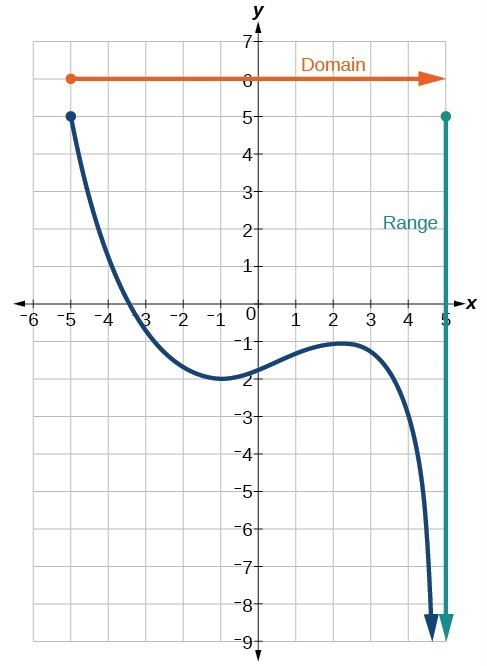So now we noticed that the cubic function graph extends horizontally from to the right without bound, so the domain is [−5,∞)

The vertical of the graph is all range values 5and below, so the range is 0 (−∞,5].

Finally, the domain and the range are forever from smaller to larger values, or from left to right for the domain, and from the bottom of the graph to the top of the graph for range of the cubic function.

## Inverse of Cubic function

A step by step interactive worksheet to be used to develop the skill of finding the inverse of cubic function is presented.

### Step by step solution

STEP 1: Note the cubic function as an equation replacing f (x) by y.

STEP 2: Solve the cubic function obtained in step 1.

STEP 3:  Interchange x and y in the above equation.

STEP 4: Write the inverse cubic function.

 Example

Find the inverse of cubic function?

$$f(x) =\frac{1}{2}(\frac{1}{2}x + 3)^3 - 1$$

Solution:

Step 1 Note the function as a cubic function replacing f(x) with y.

STEP 2 Solve for the equation obtained in step 1.

\begin{align}+2y+2 &= (\frac{1}{2}x+3)^3\\\frac{1}{2} x+3 &= 3\sqrt{+2y+2}\\x &= +2 ( 3\sqrt{+2y+2 −3})\end{align}

STEP 3 Interchange x and y in the above equation.

$Y = +2 (\sqrt{+2x+2 − 3})$

STEP 4 Write the inverse cubic function.

$f^{−1} (x) = y = +2 (3\sqrt{+2x +2 - 3})$

## Cubic function graph

One more method to find out the cubic function is graphical.

How to graph cubic functions by plotting points?

Typically, a cubic function is $$y = ax^3 + bx + cx + d$$ where a, b, c, and d are real numbers and a is not zero.

Now, We can plot points graphically.

 Example

Draw the graph of cubic function.

Graph $$y = x^3 + 3 \text{ for } –3 ≤ x ≤ 3$$ and find:

a) The value of y then x = 5

b) The value of x then y = –15

Solution:

 x –3 –2 –1 0 1 2 3 y –24 –5 2 3 4 11 30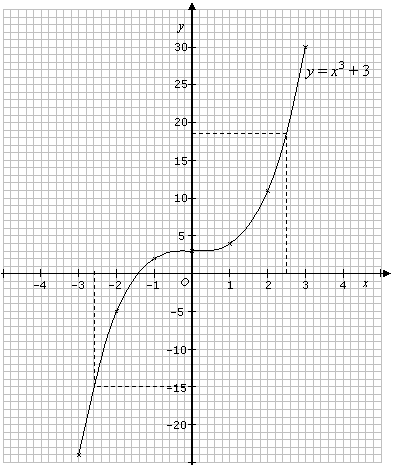a) When $$x = 2.5, y ≈ 18.6$$

b) When $$y = –15, x ≈–2.6$$

## How to Factor a Cubic Function?

A cubic polynomial is also known as a polynomial of form $$f(x)=ax^3+bx^2+cx+d, \text{where, } a ≠ 0.$$

And then the coefficients are the real numbers. The polynomial is written as the product of a linear polynomial and a quadratic polynomial.

### Existence of a Linear Factor

Algebra theorem includes that every irreducible polynomial with real coefficients is linear or quadratic, so a cubic polynomial must split as a product of two lower-degree factors. One of these conditions to be linear and the other quadratic (the quadratic might be irreducible or might itself split into a product of two linear polynomials).

A more down-to-earth way to see that every cubic polynomial has a real root (and hence a linear factor) is to notice that for large x, the lead term ax^3 dominates, so the sign of $$f(x)$$ or large positive x is the sign of a and the sign of $$f(x)$$ for large negative x is the sign of -a.

So,

F (x) is negative for some x and positive for others; by the intermediate value theorem, there must be at least one point where the graph of Y = f(x) crosses the x-axis.

### Factoring - General Case

References, mainly most of the cubic polynomials with real coefficients do not have rational roots. The difficult part of factoring a cubic polynomial, in general, is finding a real root. Once a root r is found, the cubic polynomial factors as $$f( x ) = ( x - r ) g (x),$$ where

$$G( x )$$ is the quadratic equation, and quadratic polynomials can be factored easily via the quadratic formula.

## Properties of the cubic function

We have a few properties/characteristics of the cubic function.The Degrees of three polynomials are also known as cubic polynomials. Cubic polynomials have these characteristics:

$y=ax^3+bx^2+cx+d$

• One to three roots.
• Two or zero extrema.
• One inflection point.
• Point symmetry about the inflection point.
• The range is the set of real numbers.
• Three fundamental shapes.
• Four points of the information are required to define a cubic polynomial function.
• Roots are solvable by radicals
 Number of Roots 2 1 1 1 0 1
 Example 1

$$y=x^3+3x^2-x-4$$

### Characteristics of the Cubic polynomial

• One, two, or three roots.
• Two extremes.
• One point of inflection.
• Point symmetry at the point of inflection.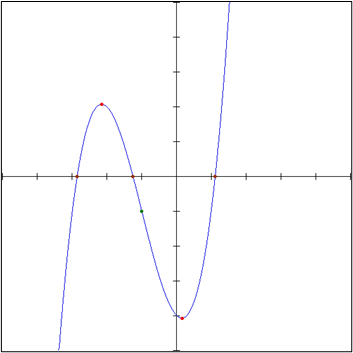Example 2

$$y=x^3+3x^2-3x-2$$

### Characteristics of the Cubic Polynomial

• One root.
• No extrema.
• One point of inflection.
• Regularly increasing or decreasing except at inflection point.
• Point symmetry at the point of inflection.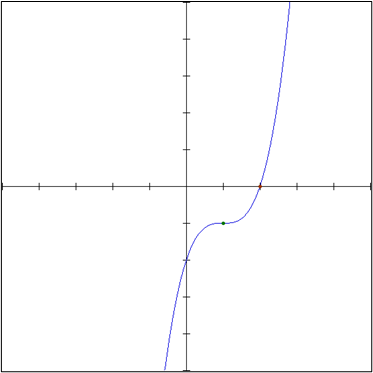Example 3

### Characteristics of the Cubic Polynomial

• One root.
• No extrema.
• One point of inflection.
• Continuously increasing or continuously decreasing.
• Point symmetry at the point of inflection.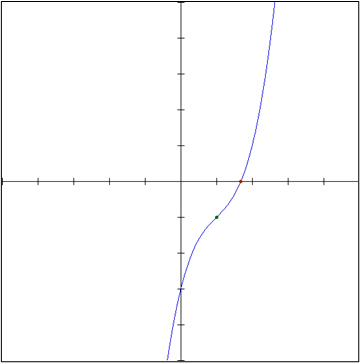## Summary

Any function of the form $$y=x^3$$ is called a cubic function or cube function.

Consider the cubic function $$f(x)=(x+d)^3+c$$

1) Then $$( c > 0 ),$$ so the graph shifts c units up ; if $$c < 0,$$ the graph shifts c units down.

2) Then $$( d > 0 ),$$ so the graph shifts d units to the left ; if $$d < 0,$$ the graph shifts d units to the right.

We saw the graph of $$x^3$$ and various other forms. We also saw how to factor a cubic function, domain and range of cubic function.

Written by Neha Tyagi

## What is the Remainder Theorem?

If a polynomial, $$f(x),$$ is divided by $$x - k,$$ the remainder is equal to $$f(k).$$

## What is the Factor Theorem?

$$x - k$$ is a factor of the polynomial $$f(x)$$ if and only if $$f(k) = 0$$

## How to solve cubic functions using the Factor Theorem?

We will consider how to solve the cubic function of the form $$px^3 + qx^2 + rx + s = 0$$ where p, q, r and s are constants by using the Factor Theorem and Synthetic Division.

Related Articles
GIVE YOUR CHILD THE CUEMATH EDGE
Access Personalised Math learning through interactive worksheets, gamified concepts and grade-wise courses